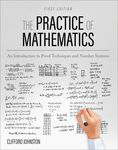## College of Arts & Sciences Faculty Books#### Title

The Practice of Mathematics: An Introduction to Proof Techniques and Number Systems

#### Description

The Practice of Mathematics: An Introduction to Proof Techniques and Number Systems is designed to help students prepare for higher-level mathematics courses through an introduction to the methods and practices of logic and proof. The book uses the development of set theory and the number systems as a framework for the introduction of the various proof techniques.
As students study proof techniques, they learn about basic set theory, natural numbers, integers, rational numbers, and real numbers. Within each chapter, ideas critical to the number systems are expanded to motivate the study of more advanced topics. In this way, students are exposed to basic ideas and concepts in modern algebra, graph theory, combinatorics, real analysis, and topology.
Additionally, the text serves as an introduction to mathematics as a profession, covering mathematical disciplines, professional activities, and mathematical software. It includes a large variety of exercises that range from easy to difficult and serve to instill key concepts and provide students with opportunities for practical application.
Emphasizing the simultaneous development of proof techniques within the content of set theory and the number systems, The Practice of Mathematics is an exemplary resource for students pursuing an undergraduate degree in mathematics.

#### ISBN

978-1-7935-0731-0

2021

San Diego, CA

#### Disciplines

Number TheoryCOinS